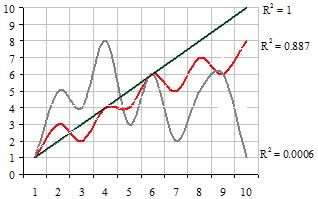# R Squared

Updated November 4, 2020

## What is R Squared?

R-squared, usually represented as R2, is a technique that evaluates the statistical relationship between two series of events. It is commonly used to describe the portion of a security's movement in the market relative to the movement of a related index.

## How Does R Squared Work?

Technically, R2 measures the proportion of variability in a dataset.  It is calculated using a statistical method that measures the proportion of the data to the expected variance of the data.  If the variance is high, the R2 is low.  Conversely, if the variance is low (i.e. the observed value is close to what would be expected), the R2 is high.  Statistically, R2 falls between 0 and 1. In financial reports, R-squared appears as a value between 0 and 100 (it is the R2 times 100.) This measure describes how well future outcomes are likely to be predicted by a statistical model.

As the illustrative graphic below shows, two events with a 1 for 1 relationship (i.e. one unit along the x axis is matched by one unit along the Y axis, the R2 is 1.  Where the movements of the two events are not closely matched, for example, the R2 falls. Where there is no apparent relationship between the events, (i.e. where the events appear random in relation to each other), the R2 falls close to 0.Practically, R2 is a useful tool in determining the correlation between events.  For example, the close relationship or correlation between prices of particular supplies can be described by R2, usually because the supplier may be in the same industry and driven by the same supply and demand factors. The R2 for fixed-income securities is measured against the T-bill. Equities are measured against the S&P 500.

## Why Does R Squared Matter?

R-squared is commonly used to summarize a statistical relationship or statistical correlation between two events.  While that may be true, it does not prove that there is a causal relationship. As with most statistical models, their predictive power is only as good as the understanding of the events themselves. In the securities markets, a high R-squared suggests that a security's performance is in-line with the movements of the index. A low R-squared suggests that the security does not follow the index.

All of our content is verified for accuracy by Paul Tracy and our team of certified financial experts. We pride ourselves on quality, research, and transparency, and we value your feedback. Below you'll find answers to some of the most common reader questions about R Squared.
##### Be the first to ask a question

Paul Tracy - 140Paul has been a respected figure in the financial markets for more than two decades. Prior to starting InvestingAnswers, Paul founded and managed one of the most influential investment research firms in America, with more than 3 million monthly readers.